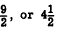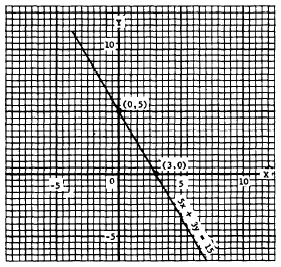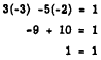X and Y InterceptsCustom SearchX AND Y INTERCEPTS Any straight line which is not parallel to one . of the axes has an X intercept and a Y intercept. These are the points at which the line crosses the X and Y axes. At the X intercept, the graph line is touching the X axis, and thus the Y value at that point is 0. At the Y intercept, the graph line is touching the Y axis; the X value at that point is 0. In order to find the X intercept, we simply let y = 0 and find the corresponding value of x. The Y intercept is found by letting x = 0 and finding the corresponding value of y. For ex- ample, the line 5x + 3y = 15 crosses the Y axis at (0,5). This may be verlfied by letting x = 0 in the equation. The X intercept is (3,0), since x is 8 when y is 0. Picture 12-3 shows the Line 5x + 3y = 15 graphed by means of the X and Y intercepts. EQUATIONS IN ONE VARIABLE An equation containing only one variable its easily graphed, since the line it represents lies parallel to an axis. For example, in 2y=9 the value of y isFigure 12-3.-Graph of 5x + 3y = 15. The line 2y = 9 lies parallel to the X axis at a distance of 4 1/2 units above it. (See fig. 12-4.) Notice that each small division on the graph paper in figure 12-4 represents one-half unit. The line 4x + 15 = 0 lies parallel to the Y axis. The value of x is - 15/4. Since this value is negative, the line lines to the left of the Y axis at a distance of 3/4 units. (See fig. 12-4.)Figure 12-4.-Graphs of 2y = 9 and 4x + I5 = 0. From the foregoing discussion, we arrive at two important conclusions: 1. A pair of numbers that satisfy an equation are the coordinates of a point on the graph of the equation. 2. The coordinates of any point on the graph of an equation will satisfy that equation. SOLVING EQUATIONS IN TWO VARIABLES A solution of a linear equation in two variables consists of a pair of numbers that satisfy the equation. For example, x = 2 and y = 1 constitute a solution of 3x - 5y = 1 When 2 is substituted for x and 1 is substituted for y, we have 3(2) - 5(1) = 1 The numbers x = -3 and y = -2 also form a solution. This is true because substituting -3 for x and -2 for y reduces the equation to an identity:Each pair of numbers (x, y) such as (2, 1) or (-3, -2) locates a point on the line 3x - 5y = 1. Many more solutions could be found. Any two numbers that constitute a solution of the equation are the coordinates of a point on the line represented by the equation. Suppose we were asked to solve a problem such as: Find two numbers such that their sum is 35 and their difference is 5. We could indicate the problem algebraically by letting x represent one number and y the other. Thus, the problem may be indicated by the two equations         x+y=33         x-y= 5 Considered separately, each of these equations represents a straight line on a graph. There are many pairs of values for x and y which satisfy the first equation, and many other pairs which satisfy the second equation. Our problem is to find ONE pair of values that will satisfy BOTH equations. Such a pair of values is said to satisfy both equations at the same time, or simultaneously. Hence, two equations for which we seek a common solution are called SIMULTANEOUS EQUATIONS. The two equations, taken together, comprise a SYSTEM of equations.Integrated Publishing, Inc. - A (SDVOSB) Service Disabled Veteran Owned Small Business